# NCERT solutions for Mathematics Exemplar Class 8 chapter 2 - Data Handling [Latest edition]

#### Chapters## Chapter 2: Data Handling

Exercise
Exercise [Pages 42 - 59]

### NCERT solutions for Mathematics Exemplar Class 8 Chapter 2 Data Handling Exercise [Pages 42 - 59]

#### Choose the correct alternative:

Exercise | Q 1 | Page 42

The height of a rectangle in a histogram shows the ______.

• Width of the class

• Upper limit of the class

• Lower limit of the class

• Frequency of the class

Exercise | Q 2 | Page 42

A geometric representation showing the relationship between a whole and its parts is a ______.

• Pie chart

• Histogram

• Bar graph

• Pictograph

Exercise | Q 3 | Page 42

In a pie chart, the total angle at the centre of the circle is ______.

• 180°

• 360°

• 270°

• 90°

Exercise | Q 4 | Page 42

The range of the data 30, 61, 55, 56, 60, 20, 26, 46, 28, 56 is ______.

• 26

• 30

• 41

• 61

Exercise | Q 5 | Page 42

Which of the following is not a random experiment?

• Tossing a coin

• Rolling a dice

• Choosing a card from a deck of 52 cards

• Throwing a stone from a roof of a building

Exercise | Q 6 | Page 42

What is the probability of choosing a vowel from the alphabets?

• 21/26

• 5/26

• 1/26

• 3/26

Exercise | Q 7 | Page 42

In a school only, 3 out of 5 students can participate in a competition. What is the probability of the students who do not make it to the competition?

• 0.65

• 0.4

• 0.45

• 0.6

Exercise | Q 8 | Page 42

Which colour received 1/5 of the votes?

• Red

• Blue

• Green

• Yellow

Exercise | Q 9 | Page 43

If 400 students voted in all, then how many did vote ‘Others’ colour as their favourite?

• 6

• 20

• 24

• 40

Exercise | Q 10 | Page 43

Which of the following is a reasonable conclusion for the given data?

• 1/20th student voted for blue colour

• Green is the least popular colour

• The number of students who voted for red colour is two times the number of students who voted for yellow colour

• Number of students liking together yellow and green colour is approximately the same as those for red colour

Exercise | Q 11 | Page 43

Listed below are the temperature in °C for 10 days. –6, –8, 0, 3, 2, 0, 1, 5, 4, 4. What is the range of the data?

• 8

• 13°C

• 10°C

• 12°C

Exercise | Q 12 | Page 43

Ram put some buttons on the table. There were 4 blue, 7 red, 3 black and 6 white buttons in all. All of a sudden, a cat jumped on the table and knocked out one button on the floor. What is the probability that the button on the floor is blue?

• 7/20

• 3/5

• 1/5

• 1/4

Exercise | Q 13 | Page 43

Rahul, Varun and Yash are playing a game of spinning a coloured wheel. Rahul wins if spinner lands on red. Varun wins if spinner lands on blue and Yash wins if it lands on green. Which of the following spinner should be used to make the game fair?

•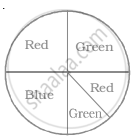•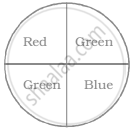•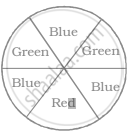•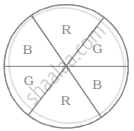Exercise | Q 14 | Page 44

In a frequency distribution with classes 0 – 10, 10 – 20 etc., the size of the class intervals is 10. The lower limit of fourth class is ______.

• 40

• 50

• 20

• 30

Exercise | Q 15 | Page 43

A coin is tossed 200 times and head appeared 120 times. The probability of getting a head in this experiment is ______.

• 2/5

• 3/5

• 1/5

• 4/5

Exercise | Q 16 | Page 44

Data collected in a survey shows that 40% of the buyers are interested in buying a particular brand of toothpaste. The central angle of the sector of the pie chart representing this information is ______.

• 120°

• 150°

• 144°

• 40°

Exercise | Q 17 | Page 43

Monthly salary of a person is Rs. 15000. The central angle of the sector representing his expenses on food and house rent on a pie chart is 60°. The amount he spends on food and house rent is ______.

• Rs. 5000

• Rs. 2500

• Rs. 6000

• Rs. 9000

Exercise | Q 18 | Page 44

The following pie chart gives the distribution of constituents in the human body. The central angle of the sector showing the distribution of protein and other constituents is ______.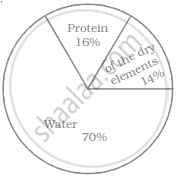• 108°

• 54°

• 30°

• 216°

Exercise | Q 19 | Page 45

Rohan and Shalu are playing with 5 cards as shown in the figure. What is the probability of Rohan picking a card without seeing, that has the number 2 on it?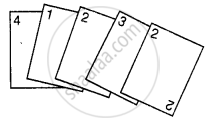• 2/5

• 1/5

• 3/5

• 4/5

Exercise | Q 20 | Page 45

The following pie chart represents the distribution of proteins in parts of a human body. What is the ratio of distribution of proteins in the muscles to that of proteins in the bones?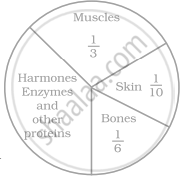• 3 : 1

• 1 : 2

• 1 : 3

• 2 : 1

Exercise | Q 21 | Page 45

What is the central angle of the sector (in the above pie chart) representing skin and bones together?

• 36°

• 60°

• 90°

• 96°

Exercise | Q 22 | Page 45

What is the central angle of the sector (in the above pie chart) representing hormones enzymes and other proteins ______.

• 120°

• 144°

• 156°

• 176°

Exercise | Q 23 | Page 45

A coin is tossed 12 times and the outcomes are observed as shown below: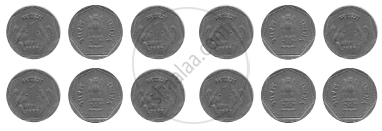The chance of occurrence of Head is ______.

• 1/2

• 5/12

• 7/12

• 5/7

Exercise | Q 24 | Page 45

Total number of outcomes, when a ball is drawn from a bag which contains 3 red, 5 black and 4 blue balls is ______.

• 8

• 7

• 9

• 12

Exercise | Q 25 | Page 45

A graph showing two sets of data simultaneously is known as ______.

• Pictograph

• Histogram

• Pie chart

• Double bar graph

Exercise | Q 26 | Page 46

Size of the class 150 –175 is ______.

• 150

• 175

• 25

• –25

Exercise | Q 27 | Page 46

In a throw of a dice, the probability of getting the number 7 is ______.

• 1/2

• 1/6

• 1

• 0

Exercise | Q 28 | Page 46

Data represented using circles is known as ______.

• Bar graph

• Histogram

• Pictograph

• Pie chart

Exercise | Q 29 | Page 46

Tally marks are used to find ______.

• Class intervals

• Range

• Frequency

• Upper limit

Exercise | Q 30 | Page 46

Upper limit of class interval 75 – 85 is ______.

• 10

• –10

• 75

• 85

Exercise | Q 31 | Page 46

Numbers 1 to 5 are written on separate slips, i.e one number on one slip and put in a box. Wahida pick a slip from the box without looking at it. What is the probability that the slip bears an odd number?

• 1/5

• 2/5

• 3/5

• 4/5

Exercise | Q 32 | Page 46

A glass jar contains 6 red, 5 green, 4 blue and 5 yellow marbles of same size. Hari takes out a marble from the jar at random. What is the probability that the chosen marble is of red colour?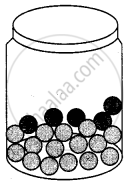• 7/10

• 3/10

• 4/5

• 2/5

Exercise | Q 33 | Page 46

A coin is tossed two times. The number of possible outcomes is ______.

• 1

• 2

• 3

• 4

Exercise | Q 34 | Page 46

A coin is tossed three times. The number of possible outcomes is ______.

• 3

• 4

• 6

• 8

Exercise | Q 35 | Page 46

A diece is tossed two times. The number of possible outcomes is ______.

• 12

• 24

• 36

• 30

#### Fill in the blanks:

Exercise | Q 36 | Page 47

Data available in an unorganised form is called ______ data.

Exercise | Q 37 | Page 47

In the class interval 20 – 30, the lower class limit is ______.

Exercise | Q 38 | Page 47

In the class interval 26 – 33, 33 is known as ______.

Exercise | Q 39 | Page 47

The range of the data 6, 8, 16, 22, 8, 20, 7, 25 is ______.

Exercise | Q 40 | Page 47

A pie chart is used to compare ______ to a whole.

Exercise | Q 41 | Page 47

In the experiment of tossing a coin one time, the outcome is either ______ or ______.

Exercise | Q 42 | Page 47

When a dice is rolled, the six possible outcomes are ______.

Exercise | Q 43 | Page 47

Each outcome or a collection of outcomes in an experiment makes an ______.

Exercise | Q 44 | Page 47

An experiment whose outcomes cannot be predicted exactly in advance is called a ______ experiment.

Exercise | Q 45 | Page 47

The difference between the upper and lower limit of a class interval is called the ______ of the class interval.

Exercise | Q 46 | Page 47

The sixth class interval for a grouped data whose first two class intervals are 10 – 15 and 15 – 20 is ______.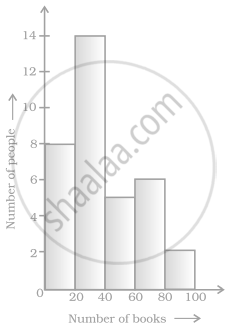Histogram given on the right shows the number of people owning the different number of books.

Exercise | Q 47 | Page 47

The total number of people surveyed is ______.

Exercise | Q 48 | Page 47

The number of people owning books more than 60 is ______.

Exercise | Q 49 | Page 47

The number of people owning books less than 40 is ______.

Exercise | Q 50 | Page 47

The number of people having books more than 20 and less than 40 is ______.

Exercise | Q 51 | Page 47

The number of times a particular observation occurs in a given data is called its ______.

Exercise | Q 52 | Page 48

When the number of observations is large, the observations are usually organised in groups of equal width called ______.

Exercise | Q 53 | Page 48

The total number of outcomes when a coin is tossed is ______.

Exercise | Q 54 | Page 48

The class size of the interval 80 – 85 is ______.

Exercise | Q 55 | Page 48

In a histogram ______ are drawn with width equal to a class interval without leaving any gap in between.

Exercise | Q 56 | Page 48

When a dice is thrown, outcomes 1, 2, 3, 4, 5, 6 are equally ______.

Exercise | Q 57 | Page 48

In a histogram, class intervals and frequencies are taken along ______ axis and ______ axis.

Exercise | Q 58 | Page 48

In the class intervals 10 – 20, 20 – 30, etc., respectively, 20 lies in the class ______.

#### State whether the following statement is True or False:

Exercise | Q 59 | Page 48

In a pie chart a whole circle is divided into sectors.

• True

• False

Exercise | Q 60 | Page 48

The central angle of a sector in a pie chart cannot be more than 180°.

• True

• False

Exercise | Q 61 | Page 48

Sum of all the central angles in a pie chart is 360°.

• True

• False

Exercise | Q 62 | Page 48

In a pie chart two central angles can be of 180°.

• True

• False

Exercise | Q 63 | Page 48

In a pie chart two or more central angles can be equal.

• True

• False

Exercise | Q 64 | Page 48

Getting a prime number on throwing a die is an event.

Using the following frequency table, answer question 65 - 68

 Marks (obtained out of 10) 4 5 7 8 9 10 Frequency 5 10 8 6 12 9
• True

• False

Exercise | Q 65 | Page 48

9 students got full marks.

• True

• False

Exercise | Q 66 | Page 48

The frequency of less than 8 marks is 29

• True

• False

Exercise | Q 67 | Page 48

The frequency of more than 8 marks is 21.

• True

• False

Exercise | Q 68 | Page 48

10 marks the highest frequency.

• True

• False

Exercise | Q 69 | Page 48

If the fifth class interval is 60 – 65, fourth class interval is 55 – 60, then the first-class interval is 45 – 50.

• True

• False

Exercise | Q 70 | Page 49

From the histogram given on the right, we can say that 1500 males above the age of 20 are literate.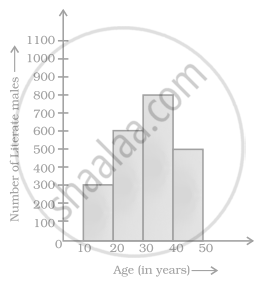• True

• False

Exercise | Q 71 | Page 49

The class size of the class interval 60 – 68 is 8.

• True

• False

Exercise | Q 72 | Page 49

If a pair of coins is tossed, then the number of outcomes are 2.

• True

• False

Exercise | Q 73 | Page 49

On throwing a dice once, the probability of occurence of an even number is 1/2.

• True

• False

Exercise | Q 74 | Page 49

On throwing a dice once, the probability of occurence of a composite number is 1/2.

• True

• False

Exercise | Q 75 | Page 49

From the given pie chart, we can infer that production of Manganese is least in state B.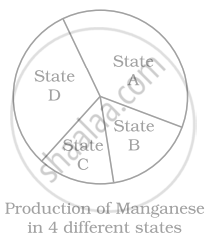• True

• False

Exercise | Q 76 | Page 49

One or more outcomes of an experiment make an event.

• True

• False

Exercise | Q 77 | Page 49

The probability of getting number 6 in a throw of a dice is 1/6. Similarly the probability of getting a number 5 is 1/5.

• True

• False

Exercise | Q 78 | Page 49

The probability of getting a prime number is the same as that of a composite number in a throw of a dice.

• True

• False

Exercise | Q 79 | Page 49

In a throw of a dice, the probability of getting an even number is the same as that of getting an odd number.

• True

• False

Exercise | Q 80 | Page 50

To verify pythagoras theorem is a random experiment.

• True

• False

Exercise | Q 81 | Page 50

The following pictorial representation of data is a histogram.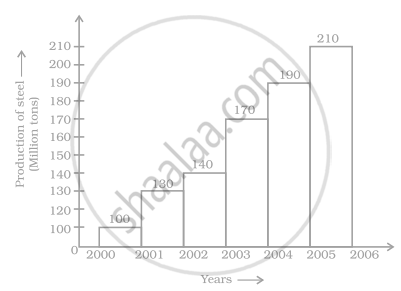• True

• False

Exercise | Q 82 | Page 50

Given below is a frequency distribution table. Read it and answer the questions that follow:

 Class Interval Frequency 10 – 20 5 20 – 30 10 30 – 40 4 40 – 50 15 50 – 60 12

(a) What is the lower limit of the second class interval?
(b) What is the upper limit of the last class interval?
(c) What is the frequency of the third class?
(d) Which interval has a frequency of 10?
(e) Which interval has the lowest frequency?
(f) What is the class size?

Exercise | Q 83 | Page 51

The top speeds of thirty different land animals have been organised into a frequency table. Draw a histogram for the given data.

 Maximum Speed (km/h) Frequency 10 – 20 5 20 – 30 5 30 – 40 10 40 – 50 8 50 – 60 0 60 – 70 2
Exercise | Q 84 | Page 51

Given below is a pie chart showing the time spend by a group of 350 children in different games. Observe it and answer the questions that follow: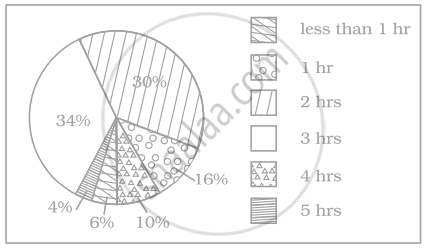(a) How many children spend at least one hour in playing games?
(b) How many children spend more than 2 hours in playing games?
(c) How many children spend 3 or lesser hours in playing games?
(d) Which is greater — number of children who spend 2 hours or more per day or number of children who play for less than one hour?

Exercise | Q 85 | Page 51

The pie chart on the right shows the result of a survey carried out to find the modes of travel used by the children to go to school. Study the pie chart and answer the questions that follow.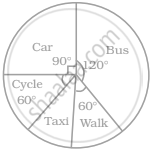(a) What is the most common mode of transport?
(b) What fraction of children travel by car?
(c) If 18 children travel by car, how many children took part in the survey?
(d) How many children use taxi to travel to school?
(e) By which two modes of transport are equal number of children travelling?

Exercise | Q 86.(a) | Page 52

A dice is rolled once. What is the probability that the number on top will be odd

Exercise | Q 86.(b) | Page 52

A dice is rolled once. What is the probability that the number on top will be greater than 5

Exercise | Q 86.(c) | Page 52

A dice is rolled once. What is the probability that the number on top will be a multiple of 3

Exercise | Q 86.(d) | Page 52

A dice is rolled once. What is the probability that the number on top will be less than 1

Exercise | Q 86.(e) | Page 52

A dice is rolled once. What is the probability that the number on top will be a factor of 36

Exercise | Q 86.(f) | Page 52

A dice is rolled once. What is the probability that the number on top will be a factor of 6

Exercise | Q 87 | Page 52

Classify the following statements under appropriate headings.
(a) Getting the sum of angles of a triangle as 180°.
(b) India winning a cricket match against Pakistan.
(c) Sun setting in the evening.
(d) Getting 7 when a die is thrown.
(e) Sun rising from the west.
(f) Winning a racing competition by you.

 Certain to happen Impossible to happen May or may not happen
Exercise | Q 88 | Page 52

Study the pie chart given below depicting the marks scored by a student in an examination out of 540. Find the marks obtained by him in each subject.

Exercise | Q 89 | Page 52

Ritwik draws a ball from a bag that contains white and yellow balls. The probability of choosing a white ball is 2/9. If the total number of balls in the bag is 36, find the number of yellow balls.

Exercise | Q 90 | Page 53

Look at the histogram below and answer the questions that follow.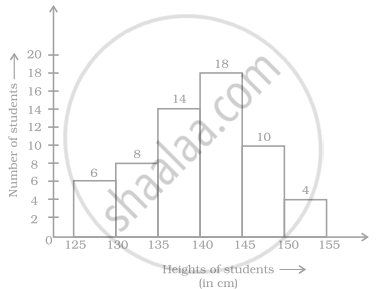(a) How many students have height more than or equal to 135 cm but less than 150 cm?
(b) Which class interval has the least number of students?
(c) What is the class size?
(d) How many students have height less than 140 cm?

Exercise | Q 91 | Page 53

Following are the number of members in 25 families of a village: 6, 8, 7, 7, 6, 5, 3, 2, 5, 6, 8, 7, 7, 4, 3, 6, 6, 6, 7, 5, 4, 3, 3, 2, 5. Prepare a frequency distribution table for the data using class intervals 0 – 2, 2 – 4, etc.

Exercise | Q 92 | Page 53

Draw a histogram to represent the frequency distribution in question 91.

Exercise | Q 93 | Page 53

The marks obtained (out of 20) by 30 students of a class in a test are as follows: 14, 16, 15, 11, 15, 14, 13, 16, 8, 10, 7, 11, 18, 15, 14, 19, 20, 7, 10, 13, 12, 14, 15, 13, 16, 17, 14, 11, 10, 20. Prepare a frequency distribution table for the above data using class intervals of equal width in which one class interval is 4 –8 (excluding 8 and including 4).

Exercise | Q 94 | Page 53

Prepare a histogram from the frequency distribution table obtained in question 93

Exercise | Q 95 | Page 54

The weights (in kg) of 30 students of a class are: 39, 38, 36, 38, 40, 42, 43, 44, 33, 33, 31, 45, 46, 38, 37, 31, 30, 39, 41, 41, 46, 36, 35, 34, 39, 43, 32, 37, 29, 26.
Prepare a frequency distribution table using one class interval as (30 – 35), 35 not included.
(i) Which class has the least frequency?
(ii) Which class has the maximum frequency?

Exercise | Q 96 | Page 54

Shoes of the following brands are sold in Nov. 2007 at a shoe store. Construct a pie chart for the data.

 Brand Number of pair of shoes sold A 130 B 120 C 90 D 40 E 20
Exercise | Q 97 | Page 54

The following pie chart depicts the expenditure of a state government under different heads.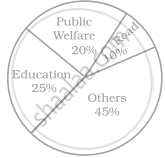(i) If the total spending is 10 crores, how much money was spent on roads?
(ii) How many times is the amount of money spent on education compared to the amount spent on roads?
(iii) What fraction of the total expenditure is spent on both roads and public welfare together?

Exercise | Q 98 | Page 54

The following data represents the different number of animals in a zoo. Prepare a pie chart for the given data.

 Animals Number of animals Deer 42 Elephant 15 Giraffe 26 Reptiles 24 Tiger 13
Exercise | Q 99.(a) | Page 55

Playing cards from a pack of cards the following cards are kept face down: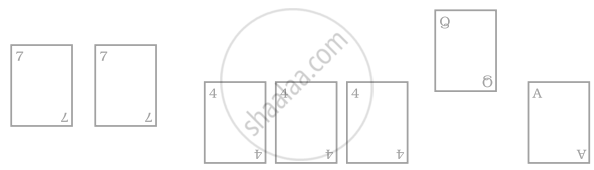Suhail wins if he picks up a face card. Find the probability of Suhail winning?

Exercise | Q 99.(b) | Page 55

Playing cards now the following cards are added to the above cards: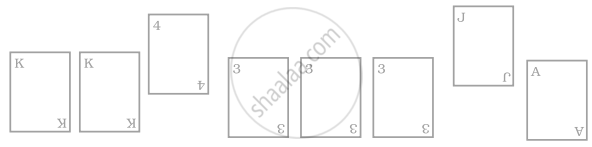What is the probability of Suhail winning now? Reshma wins if she picks up a 4. What is the probability of Reshma winning? [Queen, King and Jack cards are called face cards.]

Exercise | Q 100 | Page 55

Construct a frequency distribution table for the following weights (in grams) of 35 mangoes, using the equal class intervals, one of them is 40 – 45 (45 not included). 30, 40, 45, 32, 43, 50, 55, 62, 70, 70, 61, 62, 53, 52, 50, 42, 35, 37, 53, 55, 65, 70, 73, 74, 45, 46, 58, 59, 60, 62, 74, 34, 35, 70, 68.
(a) How many classes are there in the frequency distribution table?
(b) Which weight group has the highest frequency?

Exercise | Q 101 | Page 56

Complete the following table:

 Weights|(in kg.) Tally Marks Frequency(Number of persons) 40 – 50 |||| |||| || 50 – 60 |||| |||| |||| 60 – 70 |||| | 70 – 80 || 80 – 90 |

Find the total number of persons whose weights are given in the above table.

Exercise | Q 102 | Page 56

Draw a histogram for the following data.

 Class interval 10–15 15–20 20–25 25–30 30–35 35–40 Frequency 30 98 80 58 29 50
Exercise | Q 103 | Page 56

In a hypothetical sample of 20 people the amounts of money with them were found to be as follows:
114, 108, 100, 98, 101, 109, 117, 119, 126, 131, 136, 143, 156, 169, 182, 195, 207, 219, 235, 118.
Draw the histogram of the frequency distribution (taking one of the class intervals as 50−100).

Exercise | Q 104 | Page 56

The below histogram shows the number of literate females in the age group of 10 to 40 years in a town.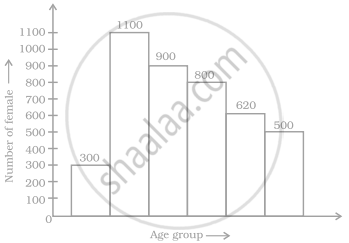(a) Write the classes assuming all the classes are of equal width.
(b) What is the classes width?
(c) In which age group are literate females the least?
(d) In which age group is the number of literate females the highest?

Exercise | Q 105 | Page 57

The following histogram shows the frequency distribution of teaching experiences of 30 teachers in various schools:
(a) What is the class width?
(b) How many teachers are having the maximum teaching experience and how many have the least teaching experience?
(c) How many teachers have teaching experience of 10 to 20 years?

Exercise | Q 106 | Page 57

In a district, the number of branches of different banks is given below:

 Bank State Bank of India Bank of Baroda Punjab National Bank Canara Bank Number of Branches 30 17 15 10

Draw a pie chart for this data.

Exercise | Q 107 | Page 57

For the development of basic infrastructure in a district, a project of Rs 108 crore approved by Development Bank is as follows:

 Item Head Road Electricity Drinking water Sewerage Amount In crore (Rs.) 43.2 16.2 27.00 21.6

Draw a pie chart for this data.

Exercise | Q 108 | Page 57

In the time table of a school, periods allotted per week to different teaching subjects are given below:

 Subject Hindi English Maths Science SocialScience Computer Sanskrit PeriodsAllotted 7 8 8 8 7 4 3

Draw a pie chart for this data.

Exercise | Q 109 | Page 57

A survey was carried out to find the favourite beverage preferred by a certain group of young people. The following pie chart shows the findings of this survey.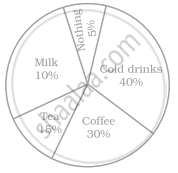From this pie chart answer the following:
(i) Which type of beverage is liked by the maximum number of people.
(ii) If 45 people like tea, how many people were surveyed?

Exercise | Q 110 | Page 58

The following data represents the approximate percentage of water in various oceans. Prepare a pie chart for the given data.

 Ocean Percentage of water Pacific 40% Atlantic 30% Indian 20% Others 10%
Exercise | Q 111.(a) | Page 58

At a Birthday Party, the children spin a wheel to get a gift. Find the probability of getting a ball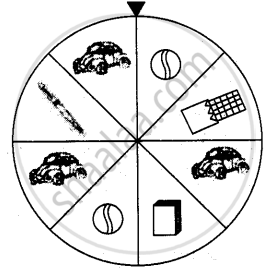Exercise | Q 111.(b) | Page 58

At a Birthday Party, the children spin a wheel to get a gift. Find the probability of getting a toy carExercise | Q 111.(c) | Page 58

At a Birthday Party, the children spin a wheel to get a gift. Find the probability of any toy except a chocolateExercise | Q 112.(a) | Page 58

Sonia picks up a card from the given cards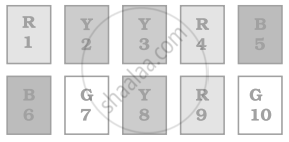Calculate the probability of getting an odd number

Exercise | Q 112.(b) | Page 58

Sonia picks up a card from the given cardsCalculate the probability of getting a Y card

Exercise | Q 112.(c) | Page 58

Sonia picks up a card from the given cardsCalculate the probability of getting a G card

Exercise | Q 112.(d) | Page 58

Sonia picks up a card from the given cardsCalculate the probability of getting B card bearing number > 7

Exercise | Q 113 | Page 58

Identify which symbol should appear in each sector in 113.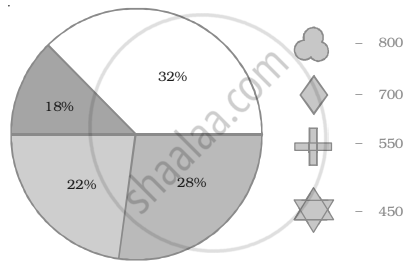Exercise | Q 114 | Page 59

Identify which symbol should appear in each sector in 114.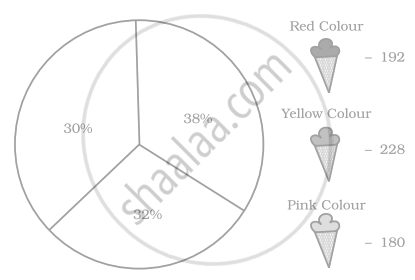Exercise | Q 115 | Page 59

A financial counselor gave a client this pie chart describing how to budget his income. If the client brings home Rs. 50,000 each month, how much should he spend in each category?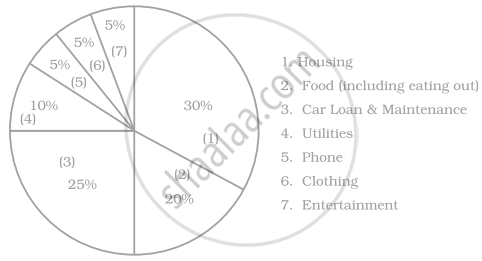Exercise | Q 116 | Page 59

Following is a pie chart showing the amount spent in rupees (in thousands) by a company on various modes of advertising for a product.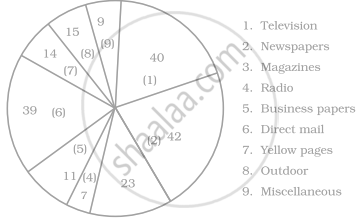1. Which type of media advertising is the greatest amount of the total?
2. Which type of media advertising is the least amount of the total?
3. What percent of the total advertising amount is spent on direct mail campaigns?
5. What media types do you think are included in miscellaneous? Why aren’t those media types given their own category?

## Chapter 2: Data Handling

Exercise## NCERT solutions for Mathematics Exemplar Class 8 chapter 2 - Data Handling

NCERT solutions for Mathematics Exemplar Class 8 chapter 2 (Data Handling) include all questions with solution and detail explanation. This will clear students doubts about any question and improve application skills while preparing for board exams. The detailed, step-by-step solutions will help you understand the concepts better and clear your confusions, if any. Shaalaa.com has the CBSE Mathematics Exemplar Class 8 solutions in a manner that help students grasp basic concepts better and faster.

Further, we at Shaalaa.com provide such solutions so that students can prepare for written exams. NCERT textbook solutions can be a core help for self-study and acts as a perfect self-help guidance for students.

Concepts covered in Mathematics Exemplar Class 8 chapter 2 Data Handling are Graphical Representation of Data as Histograms, Organisation of Data, Concept of Data Handling, Drawing a Bar Graph, Drawing a Double Bar Graph, Interpretation of a Double Bar Graph, Frequency Distribution Table, Concept of Pie Graph (Or a Circle-graph), Interpretation of Pie Diagram, Chance and Probability - Chance, Interpretation of a Pictograph, Interpretation of Bar Graphs, Basic Ideas of Probability.

Using NCERT Class 8 solutions Data Handling exercise by students are an easy way to prepare for the exams, as they involve solutions arranged chapter-wise also page wise. The questions involved in NCERT Solutions are important questions that can be asked in the final exam. Maximum students of CBSE Class 8 prefer NCERT Textbook Solutions to score more in exam.

Get the free view of chapter 2 Data Handling Class 8 extra questions for Mathematics Exemplar Class 8 and can use Shaalaa.com to keep it handy for your exam preparation